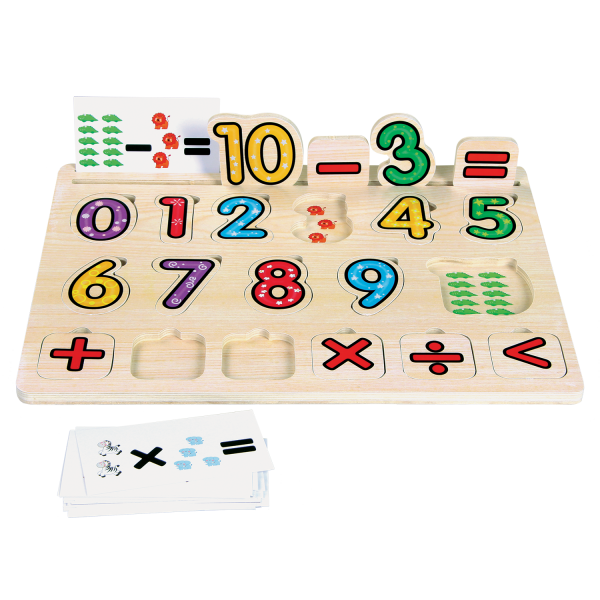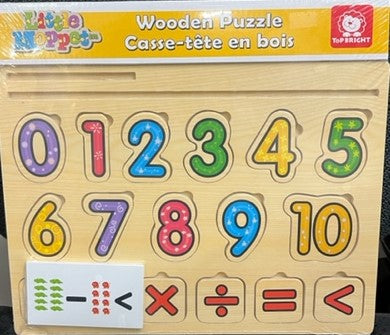# "Numbers" Wooden Puzzle with Math Equation Cards

\$ 0.00
• Great for early learning of numbers & simple math equations
• Puzzles help a child's mental development
• 10 number puzzle pieces & 6 math symbols allow children to make math equations
• Stimulates & strengthens math & language skills
• 20 additional equation cards make for more complex challenges
• For ages 5 & up